## Euler-Lagrange Differential Equation

A fundamental equation of Calculus of Variations which states that if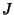is defined by an Integral of the form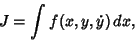(1)

where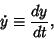(2)

thenhas a Stationary Value if the Euler-Lagrange differential equation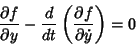(3)

is satisfied. If time Derivative Notation is replaced instead by space variable notation, the equation becomes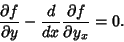(4)

In many physical problems,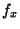(the Partial Derivative of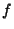with respect to) turns out to be 0, in which case a manipulation of the Euler-Lagrange differential equation reduces to the greatly simplified and partially integrated form known as the Beltrami Identity,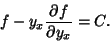(5)

For three independent variables (Arfken 1985, pp. 924-944), the equation generalizes to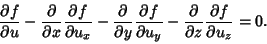(6)

Problems in the Calculus of Variations often can be solved by solution of the appropriate Euler-Lagrange equation.

To derive the Euler-Lagrange differential equation, examine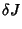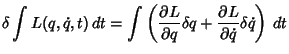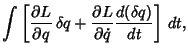(7)

since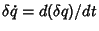. Now, integrate the second term by Parts using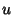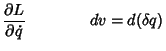(8)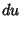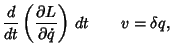(9)

so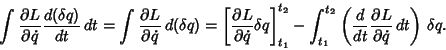(10)

Combining (7) and (10) then gives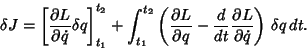(11)

But we are varying the path only, not the endpoints, so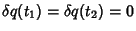and (11) becomes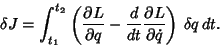(12)

We are finding the Stationary Values such that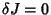. These must vanish for any small change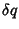, which gives from (12),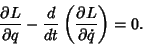(13)

This is the Euler-Lagrange differential equation.

The variation incan also be written in terms of the parameter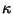as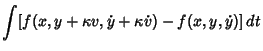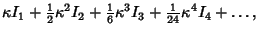(14)

where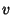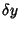(15)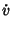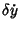(16)

and the first, second, etc., variations are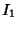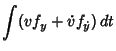(17)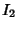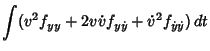(18)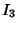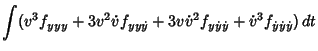(19)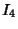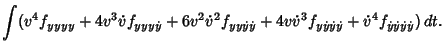(20)

The second variation can be re-expressed using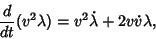(21)

so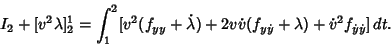(22)

But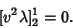(23)

Now choose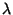such that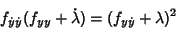(24)

and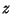such that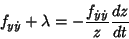(25)

so thatsatisfies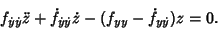(26)

It then follows that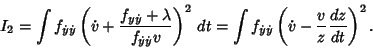(27)

References

Arfken, G. Mathematical Methods for Physicists, 3rd ed. Orlando, FL: Academic Press, 1985.

Forsyth, A. R. Calculus of Variations. New York: Dover, pp. 17-20 and 29, 1960.

Morse, P. M. and Feshbach, H. The Variational Integral and the Euler Equations.'' §3.1 in Methods of Theoretical Physics, Part I. New York: McGraw-Hill, pp. 276-280, 1953.